# Solution assignment 01 Linear functions and graphs

### Assignment 1

Draw the line: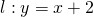and calculate the slope and the exact intersection point of the line with the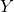-axis.

### Solution

The slope is(the coefficient of).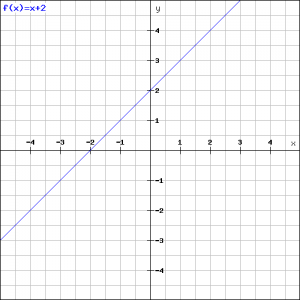The easiest way to draw the line is finding two points on the line. The line through these two points is the one.
For the first point on the line we take the point with coordinates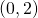. That is easy because we know that the line intersects the-axis in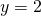.
You can find the second point by taking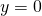and then the following linear equation has to be solved: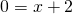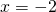and thus the coordinates of the intersection point with the-axis are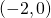. Now we can draw the line.